Next: General Dependency Networks Up: Consistent Dependency Networks Previous: Definition and Basic Properties

## Probabilistic Inference

Given a graphical model for, we often wish to answer probabilistic queries of the form
, where
(the target'' variables) and
(the input'' variables) are disjoint subsets of. This task is known in the graphical modeling community as probabilistic inference. An important special case of probabilistic inference is the determination offor given instances
of--a key component of density estimation, which has numerous applications. Given a consistent dependency network for, we can perform probabilistic inference by converting it to a Markov network, triangulating that network (forming a decomposable graphical model), and then applying one of the standard algorithms for probabilistic inference in the latter representation--for example, the junction tree algorithm of Jensen, Lauritzen, and Olesen (1990). Alternatively, we can use Gibbs sampling (e.g., Geman and Geman, 1984; Neal, 1993; Besag, Green, Higdon, and Mengersen, 1995; Gilks, Richardson, and Spiegelhalter, 1996), which we examine in some detail. First, let us consider the use of Gibbs sampling for recovering the joint distributionof a consistent dependency network for. In one simple version of Gibbs sampling, we initialize each variable to some arbitrary value. We then repeatedly cycle through each variable, in this order, and resample eachaccording to. We call this procedure an ordered Gibbs sampler. As described by the following theorem, this ordered Gibbs sampler recovers the joint distribution for.

Theorem 2: An ordered Gibbs sampler applied to a consistent dependency network for, where eachis finite (and hence discrete) and each local distribution
is positive, defines a Markov chain with a unique stationary joint distribution forequal tothat can be reached from any initial state of the chain.

Proof: Let
be the sample ofafter the
iteration of the ordered Gibbs sampler. The sequence
can be viewed as samples drawn from a homogenous Markov chain with transition matrixhaving elements
. The matrixis the product, where
is the local'' transition matrix describing the resampling of
according to the local distribution. The positivity of local distributions guarantees the positivity of, which in turn guarantees the irreducibility of the Markov chain. Consequently, there exists a unique joint distribution that is stationary with respect to. The positivity ofalso guarantees that this stationary distribution can be reached from any starting point. That this stationary distribution is equal tois proved in the Appendix.After a sufficient number of iterations, the samples in the chain will be drawn from the stationary distribution for. We use these samples to estimate. There is much written about how long to run the chain before keeping samples (the burn in'') and how many samples to use to obtain a good estimate (e.g., Gilks, Richardson, and Spiegelhalter, 1996). We do not discuss the details here. Next, let us consider the use of Gibbs sampling to computefor particular instancesandwhereandare arbitrary disjoint subsets of. A naive approach uses an ordered Gibbs sampler directly. During the iterations, only samples ofwhereare used to compute the conditional probability. An important difficulty with this approach is that if eitheroris small (a common occurrence whenorcontain many variables), many iterations are required for an accurate probability estimate. A well-known approach for estimatingwhenis small is to fixduring ordered Gibbs sampling. It is not difficult to generalize the proof of Theorem 2 to show that this modified ordered Gibbs sampler has a stationary distribution and yields the correct conditional probability given a consistent dependency network. Whenis rare becausecontains many variables, we can use the independencies encoded in a dependency network along with the law of total probability to decompose the inference task into a set of inference tasks on single variables. For example, consider the dependency network. Given the independencies specified by this network, we have
and can obtainby computing each term separately. The determination of the first term requires no Gibbs sampling--the distribution can be read directly from the local distribution forin the dependency network. The second two terms can be determined using modified ordered Gibbs samplers each with a singleton target (
and, respectively). Note that this approach has the additional advantage that some terms may be obtained by direct lookup, thereby avoiding some Gibbs sampling. In general, given a consistent dependency network forand disjoint sets of variables
and, we can obtainfor a particular instance ofandas follows:



Algorithm 1:

1
(* the unprocessed variables *)

2(* the processed and conditioning variables *)

3
(* the values for*)

4 		 While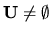5 		 		 Choosesuch thathas no more parents in
than any variable in6 		 		 If all the parents ofare in7
8 		 		 Else

9 		 		 		 Use a modified ordered Gibbs sampler to determine10
111213		 Return the product of the conditionalsThe key step in this algorithm is step 7, which bypasses Gibbs sampling when it is not needed. This step is justified by Equation 1.Next: General Dependency Networks Up: Consistent Dependency Networks Previous: Definition and Basic Properties
Journal of Machine Learning Research, 2000-10-19